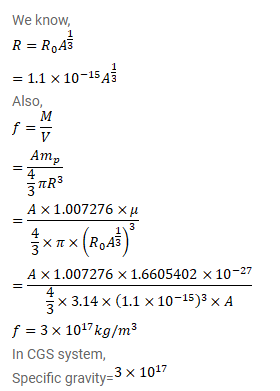# Assume that the mass of a nucleus is approximately given by

Question:

Assume that the mass of a nucleus is approximately given by $\mathrm{M}=\mathrm{Am} \mathrm{p}_{\mathrm{p}}$ where $\mathrm{A}$ is the mass number. Estimate the density of matter in $\mathrm{kg} / \mathrm{m}^{3}$ inside a nucleus. What is the specific gravity of nuclear matter?

Solution: